## Chapter 5: Linear Programming with the Simplex MethodOne of the most significant advancements in linear programming is the simplex method, developed by George Dantzig. This algorithm provides a systematic approach to finding the optimal solution to linear programming problems. In this article, we will explore the simplex method, its key concepts, and how it is applied to solve linear programming problems.

## Overview of the Linear Programming with the Simplex Method

The simplex method is a systematic approach to traverse the vertices of the polyhedron containing feasible solutions in a linear programming problem. It aims to find the optimal solution by iteratively improving the objective function value. This method is considered one of the greatest inventions of modern times due to its broad applicability in solving business-related problems.

## Formulating the Original Linear Programming Problem

To illustrate the simplex method, let’s consider a furniture production problem. We want to maximize the revenue generated by producing chairs and tables, subject to constraints on the availability of mahogany and labor. The original problem can be formulated as follows:

• Maximize Revenue = 45×1 + 80×2
• 5×1 + 20×2 ≤ 400 (Mahogany constraint)
• 10×1 + 15×2 ≤ 450 (Labor constraint)
• x1, x2 ≥ 0 (Non-negativity constraint)

In this formulation, x1 represents the number of chairs produced, x2 represents the number of tables produced, and the objective function maximizes the total revenue. The constraints limit the consumption of mahogany and labor within the available capacities.

## Transforming into Standard Form

To apply the simplex method, we transform the original problem into the standard form, which involves converting the inequalities into equalities. We introduce slack variables to represent the surplus capacity of each constraint. In this case, we add h1 and h2 as slack variables for the mahogany and labor constraints, respectively. The problem in standard form becomes:

• Maximize Revenue = 45×1 + 80×2 + 0h1 + 0h2
• 5×1 + 20×2 + h1 = 400 (Mahogany constraint)
• 10×1 + 15×2 + h2 = 450 (Labor constraint)
• x1, x2, h1, h2 ≥ 0 (Non-negativity constraint)

The slack variables h1 and h2 represent the unused capacities of mahogany and labor, respectively. We still aim to maximize the revenue while satisfying these transformed equalities.

## Basic Feasible Solutions and Canonical Form

A basic feasible solution is an initial production plan that satisfies all the constraints, with some variables set to zero. In our case, the initial solution where no chairs or tables are produced (x1=0, x2=0) represents a basic feasible solution. This solution generates zero revenue, as expected.

The non-basic variables in a basic feasible solution are set to zero, while the basic variables take positive values. In our initial solution, h1 and h2 are the basic variables, representing the unused capacities of mahogany and labor. The non-basic variables are x1 and x2, which are set to zero. This configuration is called a basic feasible solution and is an important concept in linear programming.

To express the problem in canonical form, we represent the basic variables (h1 and h2) in terms of the non-basic variables (x1 and x2). Similarly, we substitute the non-basic variables in the objective function. This process is known as pivoting. The canonical form of the problem becomes:

• Maximize z = 45×1 + 80×2 + 0h1 + 0h2
• x1 = 0 + (1/5)h1 – (4/5)h2 (Transformed mahogany constraint)
• x2 = 0 – (2/5)h1 + (3/5)h2 (Transformed labor constraint)
• x1, x2, h1, h2 ≥ 0

In the canonical form, the objective function and constraints are expressed with respect to the basic variables x1 and x2. The reduced costs associated with the non-basic variables x1 and x2 (coefficients in the objective function) indicate the potential improvement in the objective function value if these variables enter the basis.

## Iteration and Optimal Solution

In each iteration of the simplex method, we analyze the non-basic variables and their coefficients. If all the non-basic variables have coefficients ≤ 0, the current solution is optimal. Negative reduced costs associated with the non-basic variables indicate that increasing these variables would decrease the objective function value.

If there is a non-basic variable with a positive reduced cost, we choose the one with the largest coefficient to enter the basis. To determine the maximum value for this variable, we perform the minimum ratio test using the transformed equations. The minimum ratio identifies the constraint that limits the increase of the non-basic variable while staying feasible.

After selecting the entering variable, we perform pivoting to express the problem in canonical form with respect to the new basic variables. This process continues iteratively until we reach an optimal solution or determine that the problem is unbounded.

The simplex method provides a systematic approach to solving linear programming problems by iteratively improving the objective function value. By transforming the problem into the standard form and expressing it in canonical form, we can identify basic feasible solutions and optimize the objective function. The simplex method is a fundamental tool in linear programming, enabling efficient optimization in various industries and applications.

View the entire series:

• Welcome: Linear Programming Tutorial
• Chapter 1: Mathematical Programming
• Chapter 2: Introduction to Linear Programming
• Chapter 3: Mixed Integer Linear Programming Problems
• Chapter 4: Furniture Factory Problem
• Chapter 5: Simplex Method
• Chapter 6: Modeling and Solving Linear Programming Problems
• Chapter 7: Sensitivity Analysis of Linear Programming Problems
• Chapter 8: Multiple Optimal Solutions
• Chapter 9: Unbounded Linear Programming Problems
• Chapter 10: Infeasible Linear Programming Problems
• Chapter 11: Linear Programming Overview – Further Considerations
• Chapter 12: Duality in Linear Programming
• Chapter 13: Optimality Conditions
• Chapter 14: Dual Simplex Method

30 day free trial for commercial users, always free for academics.

Latest news and releases

• Linear Programming (LP) – A Primer on the Basics
• Chapter 7: Sensitivity Analysis of Linear…

Choose the evaluation license that fits you best, and start working with our Expert Team for technical guidance and support.

Request free trial hours, so you can see how quickly and easily a model can be solved on the cloud.

Looking for documentation?

## Jupyter Models

Case studies, privacy overview.

## Simplex Method for Solution of L.P.P (With Examples) | Operation Research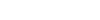After reading this article you will learn about:- 1. Introduction to the Simplex Method 2. Principle of Simplex Method 3. Computational Procedure 4. Flow Chart.

## Introduction to the Simplex Method :

Simplex method also called simplex technique or simplex algorithm was developed by G.B. Dantzeg, An American mathematician. Simplex method is suitable for solving linear programming problems with a large number of variable. The method through an iterative process progressively approaches and ultimately reaches to the maximum or minimum values of the objective function.

## Principle of Simplex Method :

It has not been possible to obtain the graphical solution to the LP problem of more than two variables. For these reasons mathematical iterative procedure known as ‘Simplex Method’ was developed. The simplex method is applicable to any problem that can be formulated in-terms of linear objective function subject to a set of linear constraints.

The simplex method provides an algorithm which is based on the fundamental theorem of linear programming. This states that “the optimal solution to a linear programming problem if it exists, always occurs at one of the corner points of the feasible solution space.”

The simplex method provides a systematic algorithm which consist of moving from one basic feasible solution to another in a prescribed manner such that the value of the objective function is improved. The procedure of jumping from vertex to the vertex is repeated. The simplex algorithm is an iterative procedure for solving LP problems.

It consists of:

(i) Having a trial basic feasible solution to constraints equation,

(ii) Testing whether it is an optimal solution,

(iii) Improving the first trial solution by repeating the process till an optimal solution is obtained.

## Computational Procedure of Simplex Method :

The computational aspect of the simplex procedure is best explained by a simple example.

Consider the linear programming problem:

Maximize z = 3x 1 + 2x 2

Subject to x 1 + x 2 , ≤ 4

x 1 – x 2 , ≤ 2

x 1 , x 2 , ≥ 4

< 2 x v x 2 > 0

The steps in simplex algorithm are as follows:

Formulation of the mathematical model:

(i) Formulate the mathematical model of given LPP.

(ii) If objective function is of minimisation type then convert it into one of maximisation by following relationship

Minimise Z = – Maximise Z*

When Z* = -Z

(iii) Ensure all b i values [all the right side constants of constraints] are positive. If not, it can be changed into positive value on multiplying both side of the constraints by-1.

In this example, all the b i (height side constants) are already positive.

(iv) Next convert the inequality constraints to equation by introducing the non-negative slack or surplus variable. The coefficients of slack or surplus variables are zero in the objective function.

In this example, the inequality constraints being ‘≤’ only slack variables s 1 and s 2 are needed.

Therefore given problem now becomes:The first row in table indicates the coefficient c j of variables in objective function, which remain same in successive tables. These values represent cost or profit per unit of objective function of each of the variables.

The second row gives major column headings for the simple table. Column C B gives the coefficients of the current basic variables in the objective function. Column x B gives the current values of the corresponding variables in the basic.

Number a ij represent the rate at which resource (i- 1, 2- m) is consumed by each unit of an activity j (j = 1,2 … n).

The values z j represents the amount by which the value of objective function Z would be decreased or increased if one unit of given variable is added to the new solution.

It should be remembered that values of non-basic variables are always zero at each iteration.

So x 1 = x 2 = 0 here, column x B gives the values of basic variables in the first column.

So 5, = 4, s 2 = 2, here; The complete starting feasible solution can be immediately read from table 2 as s 1 = 4, s 2 , x, = 0, x 2 = 0 and the value of the objective function is zero.## Flow Chart of Simplex Method :• StumbleUpon

## Output Type

Output width, output height.

To embed this widget in a post, install the Wolfram|Alpha Widget Shortcode Plugin and copy and paste the shortcode above into the HTML source.

To embed a widget in your blog's sidebar, install the Wolfram|Alpha Widget Sidebar Plugin , and copy and paste the Widget ID below into the "id" field:

## Save to My Widgets

Build a new widget.

We appreciate your interest in Wolfram|Alpha and will be in touch soon.

• Math Article

## Linear Programming

In Mathematics, linear programming is a method of optimising operations with some constraints. The main objective of linear programming is to maximize or minimize the numerical value. It consists of linear functions which are subjected to the constraints in the form of linear equations or in the form of inequalities.  Linear programming is considered an important technique that is used to find the optimum resource utilisation. The term “linear programming” consists of two words as linear and programming. The word “linear” defines the relationship between multiple variables with degree one. The word “programming” defines the process of selecting the best solution from various alternatives.

Linear Programming is widely used in Mathematics and some other fields such as economics, business, telecommunication, and manufacturing fields. In this article, let us discuss the definition of linear programming, its components, and different methods to solve linear programming problems.

• Characteristics
• Linear programming Problems
• Simplex Method

## Graphical Method

• Applications
• Practice Problems

## What is Linear Programming?

Linear programming (LP)   or Linear Optimisation may be defined as the problem of maximizing or minimizing a linear function that is subjected to linear constraints. The constraints may be equalities or inequalities. The optimisation problems involve the calculation of profit and loss.   Linear programming problems  are an important class of optimisation problems, that helps to find the feasible region and optimise the solution in order to have the highest or lowest value of the function.

In other words, linear programming is considered as an optimization method to maximize or minimize the objective function of the given mathematical model with the set of some requirements which are represented in the linear relationship. The main aim of the linear programming problem is to find the optimal solution.

Linear programming is the method of considering different inequalities relevant to a situation and calculating the best value that is required to be obtained in those conditions.  Some of the assumptions taken while working with linear programming are:

• The number of constraints should be expressed in the quantitative terms
• The relationship between the constraints and the objective function should be linear
• The linear function (i.e., objective function) is to be optimised## Components of Linear Programming

The basic components of the LP are as follows:

• Decision Variables
• Constraints
• Objective Functions

## Characteristics of Linear Programming

The following are the five characteristics of the linear programming problem:

Constraints – The limitations should be expressed in the mathematical form, regarding the resource.

Objective Function – In a problem, the objective function should be specified in a quantitative way.

Linearity – The relationship between two or more variables in the function must be linear. It means that the degree of the variable is one.

Finiteness –  There should be finite and infinite input and output numbers. In case, if the function has infinite factors, the optimal solution is not feasible.

Non-negativity – The variable value should be positive or zero. It should not be a negative value.

Decision Variables – The decision variable will decide the output. It gives the ultimate solution of the problem. For any problem, the first step is to identify the decision variables.

## Linear Programming Problems

The Linear Programming Problems (LPP) is a problem that is concerned with finding the optimal value of the given linear function. The optimal value can be either maximum value or minimum value. Here, the given linear function is considered an objective function. The objective function can contain several variables, which are subjected to the conditions and it has to satisfy the set of linear inequalities called linear constraints. The linear programming problems can be used to get the optimal solution for the following scenarios, such as manufacturing problems, diet problems, transportation problems, allocation problems and so on.

## Methods to Solve Linear Programming Problems

The linear programming problem can be solved using different methods, such as the graphical method, simplex method, or by using tools such as R, open solver etc. Here, we will discuss the two most important techniques called the simplex method and graphical method in detail.

## Linear Programming Simplex Method

The simplex method is one of the most popular methods to solve linear programming problems. It is an iterative process to get the feasible optimal solution. In this method, the value of the basic variable keeps transforming to obtain the maximum value for the objective function. The algorithm for linear programming simplex method is provided below:

Step 1 : Establish a given problem. (i.e.,) write the inequality constraints and objective function.

Step 2: Convert the given inequalities to equations by adding the slack variable to each inequality expression.

Step 3 : Create the initial simplex tableau. Write the objective function at the bottom row. Here, each inequality constraint appears in its own row. Now, we can represent the problem in the form of an augmented matrix, which is called the initial simplex tableau.

Step 4 : Identify the greatest negative entry in the bottom row, which helps to identify the pivot column. The greatest negative entry in the bottom row defines the largest coefficient in the objective function, which will help us to increase the value of the objective function as fastest as possible.

Step 5 : Compute the quotients. To calculate the quotient, we need to divide the entries in the far right column by the entries in the first column, excluding the bottom row. The smallest quotient identifies the row. The row identified in this step and the element identified in the step will be taken as the pivot element.

Step 6: Carry out pivoting to make all other entries in column is zero.

Step 7: If there are no negative entries in the bottom row, end the process. Otherwise, start from step 4.

Step 8: Finally, determine the solution associated with the final simplex tableau.

The graphical method is used to optimize the two-variable linear programming. If the problem has two decision variables, a graphical method is the best method to find the optimal solution. In this method, the set of inequalities are subjected to constraints. Then the inequalities are plotted in the XY plane. Once, all the inequalities are plotted in the XY graph, the intersecting region will help to decide the feasible region. The feasible region will provide the optimal solution as well as explains what all values our model can take.  Let us see an example here and understand the concept of linear programming in a better way.

Calculate the maximal and minimal value of z = 5x + 3y for the following constraints.

x + 2y ≤ 14

3x – y ≥ 0

x – y ≤ 2

The three inequalities indicate the constraints. The area of the plane that will be marked is the feasible region.

The optimisation equation (z) = 5x + 3y. You have to find the (x,y) corner points that give the largest and smallest values of z.

To begin with, first solve each inequality.

x + 2y ≤ 14 ⇒  y ≤ -(1/2)x + 7

3x – y ≥ 0 ⇒  y ≤ 3x

x – y ≤ 2 ⇒ y  ≥ x – 2

Here is the graph for the above equations.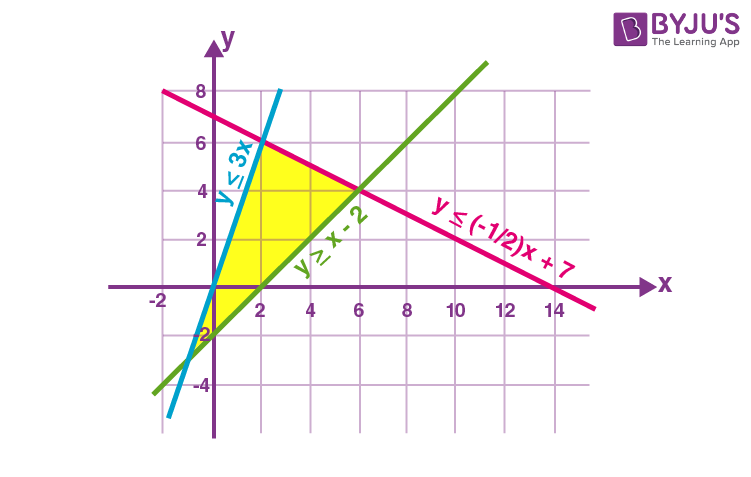Now pair the lines to form a system of linear equations to find the corner points.

y = -(½) x + 7

Solving the above equations, we get the corner points as (2, 6)

y = -1/2 x + 7

y = x – 2

Solving the above equations, we get the corner points as (6, 4)

Solving the above equations, we get the corner points as (-1, -3)

For linear systems, the maximum and minimum values of the optimisation equation lie on the corners of the feasibility region. Therefore, to find the optimum solution, you only need to plug these three points in z = 3x + 4y

z = 5(2) + 3(6) = 10 + 18 = 28

z = 5(6) + 3(4) = 30 + 12 = 42

z = 5(-1) + 3(-3) = -5 -9 = -14

Hence, the maximum of z = 42 lies at (6, 4) and the minimum of z = -14 lies at (-1, -3)

## Linear Programming Applications

A real-time example would be considering the limitations of labours and materials and finding the best production levels for maximum profit in particular circumstances. It is part of a vital area of mathematics known as optimisation techniques. The applications of LP in some other fields are

• Engineering – It solves design and manufacturing problems as it is helpful for doing shape optimisation
• Efficient Manufacturing – To maximise profit, companies use linear expressions
• Energy Industry – It provides methods to optimise the electric power system.
• Transportation Optimisation – For cost and time efficiency.

## Importance of Linear Programming

Linear programming is broadly applied in the field of optimisation for many reasons. Many functional problems in operations analysis can be represented as linear programming problems. Some special problems of linear programming are such as network flow queries and multi-commodity flow queries are deemed to be important to have produced much research on functional algorithms for their solution.

## Linear Programming Video Lesson

Linear programming problem.## Linear Programming Practice Problems

Solve the following linear programming problems:

• A doctor wishes to mix two types of foods in such a way that the vitamin contents of the mixture contain at least 8 units of vitamin A and 10 units of vitamin C. Food ‘I’ contains 2 units/kg of vitamin A and 1 unit/kg of vitamin C. Food ‘II’ contains 1 unit/kg of vitamin A and 2 units/kg of vitamin C. It costs Rs 50 per kg to purchase Food ‘I’ and Rs 70 per kg to purchase Food ‘II’. Formulate this problem as a linear programming problem to minimise the cost of such a mixture
• One kind of cake requires 200g of flour and 25g of fat, and another kind of cake requires 100g of flour and 50g of fat.  Formulate this problem as a linear programming problem to find the maximum number of cakes that can be made from 5kg of flour and 1 kg of fat assuming that there is no shortage of the other ingredients used in making the cakes.

## Frequently Asked Questions on Linear Programming

Linear programming is a process of optimising the problems which are subjected to certain constraints. It means that it is the process of maximising or minimizing the linear functions under linear inequality constraints. The problem of solving linear programs is considered as the easiest one.

## Mention the different types of linear programming.

The different types of linear programming are: Solving linear programming by Simplex method Solving linear programming using R Solving linear programming by graphical method Solving linear programming with the use of an open solver.

## What are the requirements of linear programming?

The five basic requirements of linear programming are: Objective function Constraints Linearity Non-negativity Finiteness

## Mention the advantages of Linear programming

The advantages of linear programming are: Linear programming provides insights to the business problems It helps to solve multi-dimensional problems According to the condition change, LP helps in making the adjustments By calculating the cost and profit of various things, LP helps to take the best optimal solution

## What is meant by linear programming problems?

The linear programming problems (LPP) helps to find the best optimal solution of a linear function (also, known as the objective function) which are placed under certain constraints (set of linear inequality constraints)

To learn all concepts in Maths in a more engaging way, register at BYJU’S. Also, watch interesting videos on various Maths topics by downloading BYJU’S– The Learning App.

Request OTP on Voice Call

Post My Comment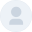Thank you so much for clearly explained notes. I benefited a lot from them

Thank you very much for this material.• Share Share

Register with byju's & watch live videos.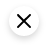• News & Events

## CSCA 5424: Approximation Algorithms and Linear Programming

Preview this course in the non-credit experience today!  Start working toward program admission and requirements right away. Work you complete in the non-credit experience will transfer to the for-credit experience when you upgrade and pay tuition. See How It Works for details.

• Course Type: Pathway | Breadth
• Specialization: Foundations of Data Structures and Algorithms
• Instructor:  Dr. Sriram Sankaranarayanan, Professor of Computer Science
• Prior knowledge needed:  You must understand all concepts covered in Dr. Sankaranarayanan's non-credit   Algorithms for Searching, Sorting, and Indexing  and  Trees and Graphs: Basics  courses to succeed in this course. We highly recommend successfully completing those two courses in the non-credit experience before starting this course; they are a great option to refresh your skills and ensure you're ready. Note that you  cannot  apply credit from either of these courses toward MS-CS graduation requirements. Calculus, probability theory: distributions, expectations and moments. Some programming experience with Python also recommended.View on Coursera

## Learning Outcomes

• Formulate linear and integer programming problems for solving commonly encountered optimization problems.
• Understand how approximation algorithms compute solutions that are guaranteed to be within some constant factor of the optimal solution.
• Develop a basic understanding of how linear and integer programming problems are solved.

## Course Content

Duration: 4 hours

This module introduces the basics of linear programs and shows how some algorithm problems (such as the network flow problem) can be posed as a linear program. We will provide hands-on tutorials on how to pose and solve a linear programming problem in Python. Finally, we will provide a brief overview of linear programming algorithms including the famous Simplex algorithm for solving linear programs. The problem set will guide you towards posing and solving some interesting problems such as a financial portfolio problem and the optimal transportation problem as linear programs.

This module will cover integer linear programming and its use in solving NP-hard (combinatorial optimization) problems. We will cover some examples of what integer linear programming is by formulating problems such as Knapsack, Vertex Cover and Graph Coloring. Next, we will study the concept of integrality gap and look at the special case of integrality gap for vertex cover problems. We will conclude with a tutorial on formulating and solving integer linear programs using the python library Pulp.

Duration: 6 hours

We will introduce approximation algorithms for solving NP-hard problems. These algorithms are fast (often greedy algorithms) that may not produce an optimal solution but guarantees that its solution is not "too far away" from the best possible. We will present some of these algorithms starting from a basic introduction to the concepts involved followed by a series of approximation algorithms for scheduling problems, vertex cover problem and the maximum satisfiability problem.

Duration: 3 hours

We will present the travelling salesperson problem (TSP): a very important and widely applicable combinatorial optimization problem, its NP-hardness and the hardness of approximating a general TSP with a constant factor. We present integer linear programming formulation and a simple yet elegant dynamic programming algorithm. We will present a 3/2 factor approximation algorithm by Christofides and discuss some heuristic approaches for solving TSPs. We will conclude by presenting approximation schemes for the knapsack problem.

Duration: 1.5 hours

This module contains materials for the final exam. If you've upgraded to the for-credit version of this course, please make sure you review the additional for-credit materials in the introductory module and anywhere else they may be found.• Cross-listed Courses: Courses that are offered under two or more programs. Considered equivalent when evaluating progress toward degree requirements. You may not earn credit for more than one version of a cross-listed course.
• Page Updates: This page is periodically updated. Course information on the Coursera platform supersedes the information on this page. Click the  View on Coursera  button above for the most up-to-date information.
• Bachelor of Science Post-Baccalaureate
• How It Works
• Student Handbook
• Current Students

Enroll in the For-Credit Experience

Apply   Visit   Give

## Departments

• Ann and H.J. Smead Aerospace Engineering Sciences
• Chemical & Biological Engineering
• Civil, Environmental & Architectural Engineering
• Computer Science
• Electrical, Computer & Energy Engineering
• Paul M. Rady Mechanical Engineering
• Applied Mathematics
• Biomedical Engineering
• Creative Technology & Design
• Engineering Management
• Engineering Physics
• Integrated Design Engineering
• Environmental Engineering
• Materials Science & Engineering

## Affiliates & Partners

• ATLAS Institute
• BOLD Center
• Discovery Learning
• Engineering Honors
• Entrepreneurship
• Herbst Program for Engineering, Ethics & Society
• Integrated Teaching and Learning
• Global Engineering
• Mortenson Center for Global Engineering
• National Center for Women & Information Technology## Solving Linear Programming Problems Using Simplex Method

To understand this subject matter first lets have a look at linear programming problems using the simplex algorithm. For those who don’t know what the simplex algorithm is let me give you a quick background. This simplex algorithm is a way of solving linear programming problems by taking a set of inputs and transforming them into another set of outputs. The main motivation behind the use of the simplex algorithm is that it makes the solving of linear problems much easier because it essentially simplifies everything.

One of the problems with linear programming is that there are usually too many factors to take into account when solving it. So when solving linear programming problems often we have to take into account such things as; time, input variables, output variables, average values and so on. In order to solve these problems effectively we need to keep track of the results of each step we make. To do this manually would be very tedious and probably impossible. Luckily we can solve linear programming problems using simplex programming assignment help.

We can solve linear programming problems by using the simplex algorithm but how can we get these kind of answers? Well if we want to use the simplex algorithm correctly, we first need to learn how to use the simplex software. The software is available online and is written in Java, which is one of the simplest languages to use and understand. The basic usage of the simplex software is to solve linear equations and the assignment to help guide us through the whole process.

Before using the software we will need to know a few details about linear equations. The first thing we should know is that every linear equation has an associated function called the basis function, and depending on the type of equation there will also be a range of unknowns called the range. The function and the range are using to solve the equation so that we can get the best possible answer. The next thing we need to do is to define a few variables for our linear programming problem and then we can start solving.

Let us start with solving linear equations. We can use the Java Simplex language so that the equation will be written in Java. We should include a main variable called a solution variable so that we can get the initial value before our solving the linear function. Next we should create two arrays that will hold the starting value of the x and the y coordinates of the point and the final value that will be the end result after the function is done. Then we can solve for both the x and y by using the formula Solving Linear Programming Problems Using Simplex Method. We can use one or two arrays for both the x and y.

Another important thing we should learn is how to solve quadratic equations. Quadratic function of a polynomial equation can be solved using the same method as we used for the linear function. We should define a few variables for our problem and then we can solve using the following code:

Simplex method was created to make it easier for people to solve almost any problems. You can find more information on solving linear and quadratic equations using this method on the internet. If you do not have the time to read books or tutorials, you can use the software that will solve these problems for you in minutes. Once you are done with the problems, you will be able to solve your problems easily using the software.#### IMAGES

1. Solved Use the simplex method to solve the linear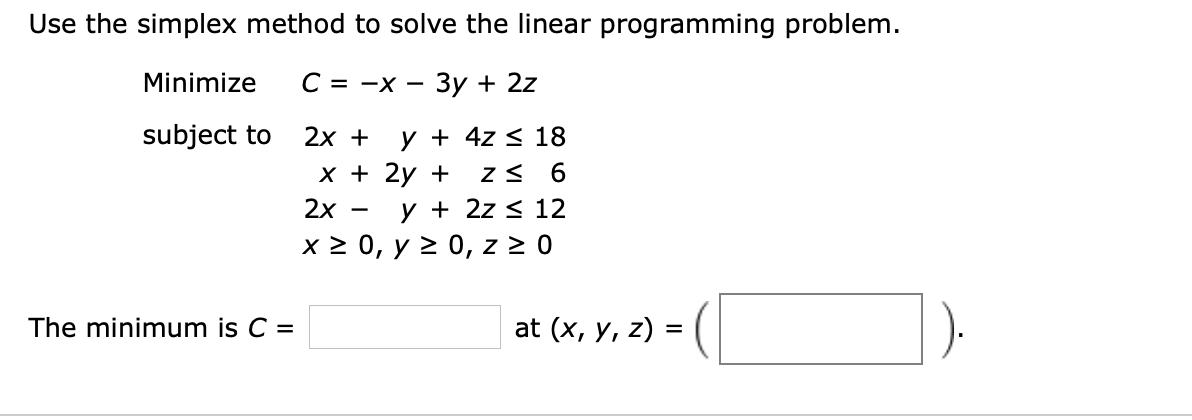2. Linear Programming-Simplex Method Standard Maximization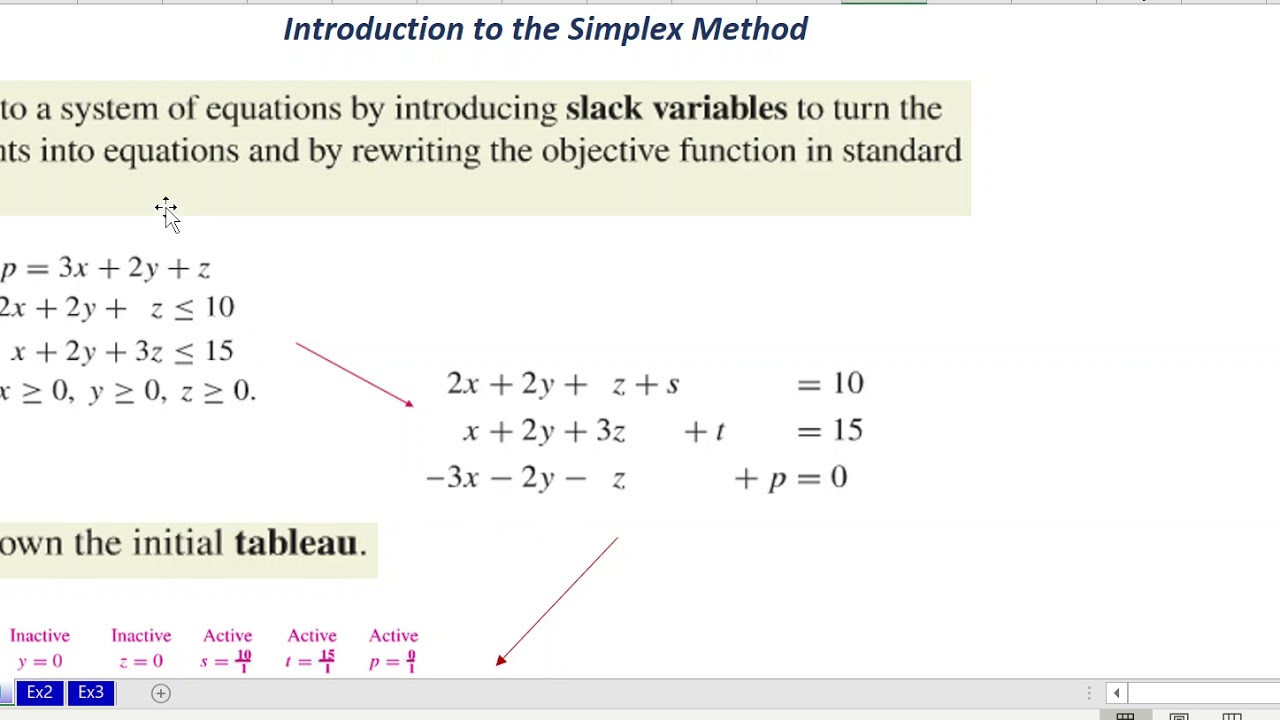3. Solved Solve the linear programming problem by the simplex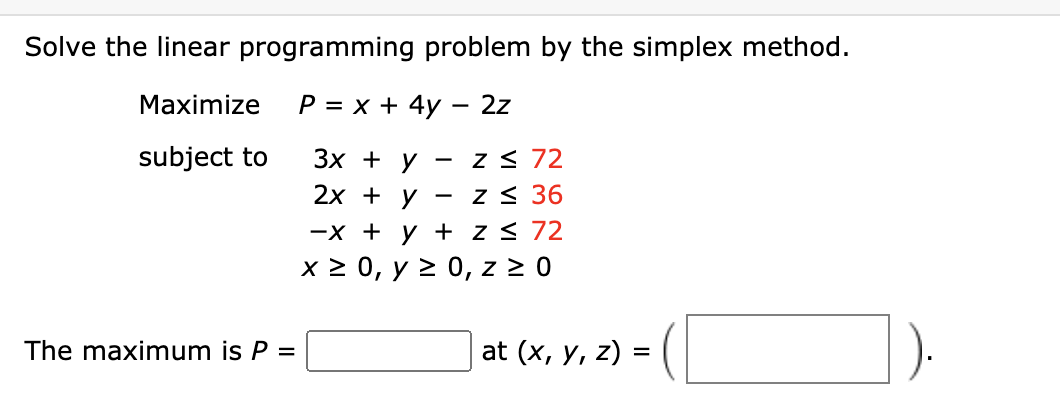4. PPT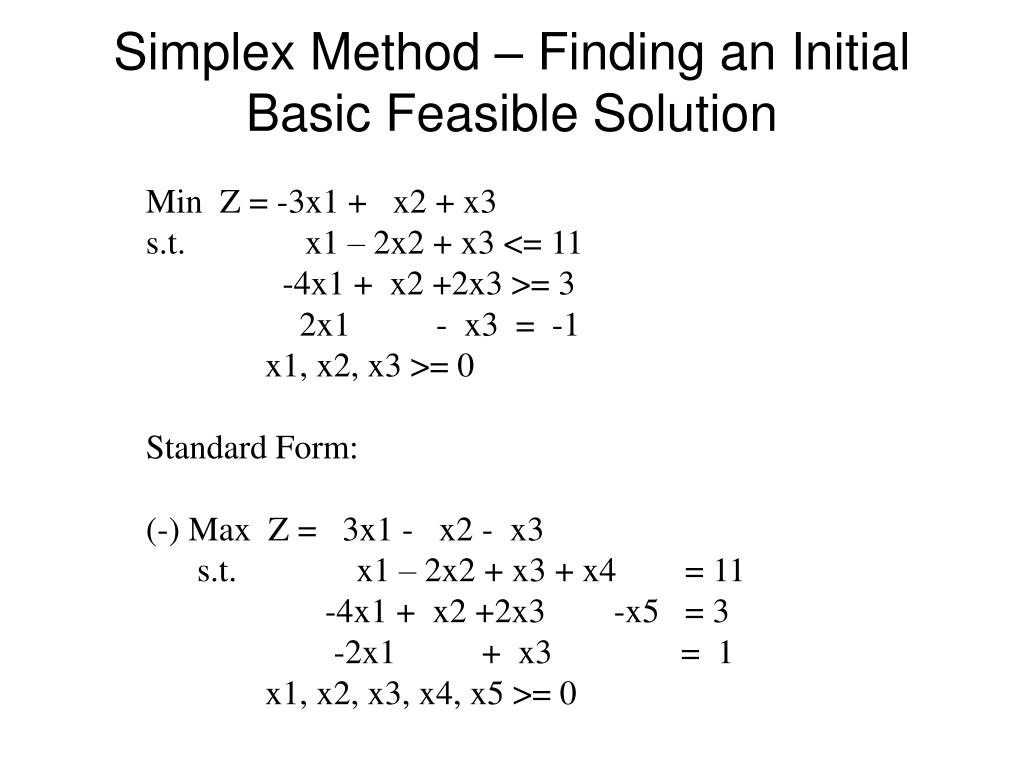5. PPT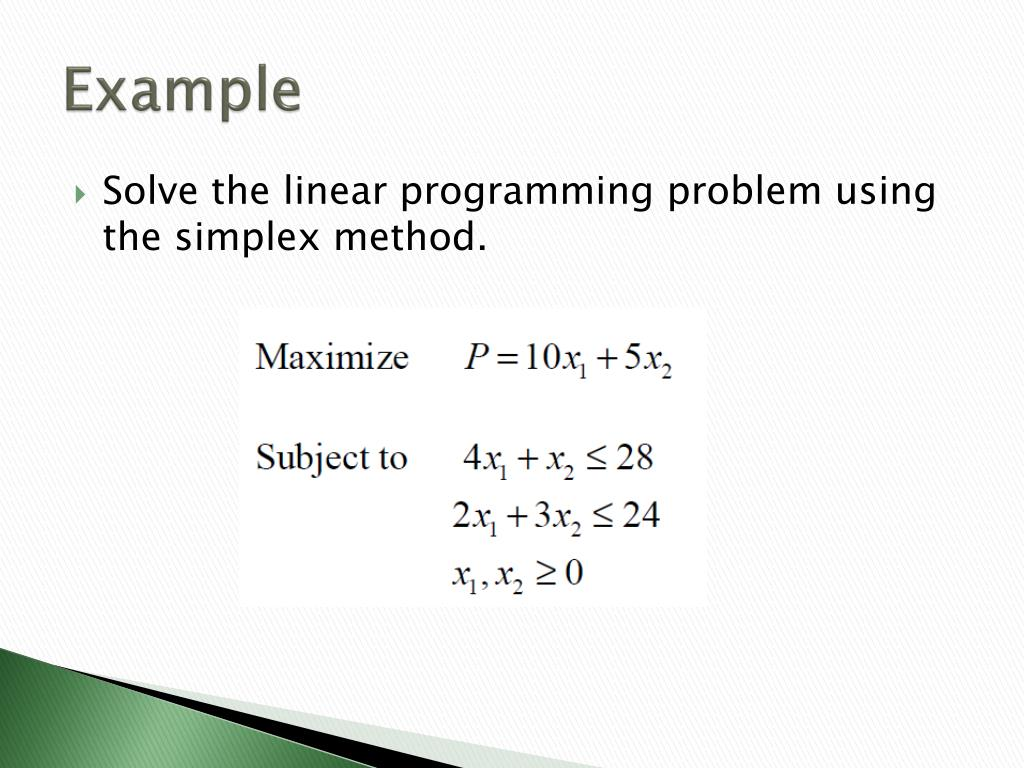6. PPT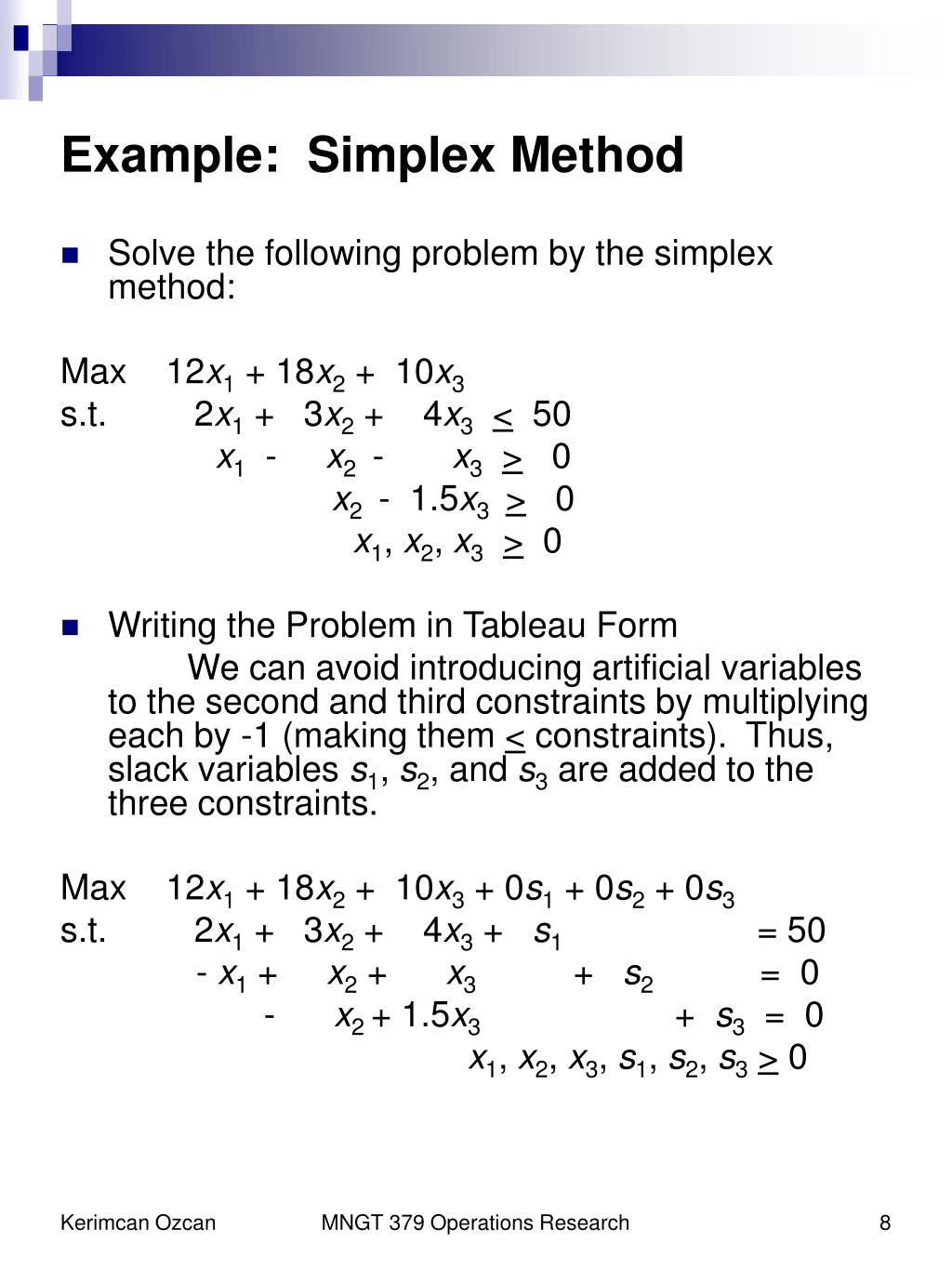1. 4.2.1: Maximization By The Simplex Method (Exercises)

SECTION 4.2 PROBLEM SET: MAXIMIZATION BY THE SIMPLEX METHOD. Solve the following linear programming problems using the simplex method. 4) A factory manufactures chairs, tables and bookcases each requiring the use of three operations: Cutting, Assembly, and Finishing. The first operation can be used at most 600 hours; the second at most 500 ...

2. 4.2: Maximization By The Simplex Method

In solving this problem, we will follow the algorithm listed above. STEP 1. Set up the problem. Write the objective function and the constraints. Since the simplex method is used for problems that consist of many variables, it is not practical to use the variables x, y, z etc. We use symbols x1, x2, x3, and so on. Let.

3. PDF Chapter 6Linear Programming: The Simplex Method

Roughly speaking, the idea of the simplex method is to represent an LP problem as a system of linear equations, and then a certain solu- tion (possessing some properties we will de ne later) of the obtained system would be an optimal solution of the initial LP problem (if any exists).

4. 4: Linear Programming

4.3: Minimization By The Simplex Method. In this section, we will solve the standard linear programming minimization problems using the simplex method. The procedure to solve these problems involves solving an associated problem called the dual problem. The solution of the dual problem is used to find the solution of the original problem.

5. PDF Lecture 6 Simplex method for linear programming

Simplex method for linear programming slides credit: Weinan E Outline Examples and standard form Fundamental theorem Simplex algorithm Example: Transportation problem Schematics of transportation problem Example: Transportation problem Formulation: m n min s = X X cijxij i=1 j=1 subject to the constraint xij ≥ bj, j = 1, . . . , n i=1

6. PDF Linear Programming: Chapter 2 The Simplex Method

Linear Programming: Chapter 2 The Simplex Method Robert J. Vanderbei October 17, 2007 ... This is how we detect unboundedness with the simplex method. Initialization Consider the following problem: maximize 3x 1 + 4x 2 subject to 4x 1 2x 2 8 2x 1 2 3x 1 + 2x 2 10 x 1 + 3x 2 1 3x 2 2 x 1;x

7. Chapter 5: Linear Programming with the Simplex Method

The simplex method provides a systematic approach to solving linear programming problems by iteratively improving the objective function value. By transforming the problem into the standard form and expressing it in canonical form, we can identify basic feasible solutions and optimize the objective function.

8. Simplex method

simplex method, standard technique in linear programming for solving an optimization problem, typically one involving a function and several constraints expressed as inequalities. The inequalities define a polygonal region, and the solution is typically at one of the vertices.

9. 3.4: Simplex Method

In this section we will explore the traditional by-hand method for solving linear programming problems. To handle linear programming problems that contain upwards of two variables, mathematicians developed what is now known as the simplex method. It is an efficient algorithm (set of mechanical steps) that "toggles" through corner points ...

10. Simplex algorithm

Overview A system of linear inequalities defines a polytope as a feasible region. The simplex algorithm begins at a starting vertex and moves along the edges of the polytope until it reaches the vertex of the optimal solution. Polyhedron of simplex algorithm in 3D The simplex algorithm operates on linear programs in the canonical form maximize

11. Simplex Method for Solution of L.P.P (With Examples)

Simplex method is suitable for solving linear programming problems with a large number of variable. The method through an iterative process progressively approaches and ultimately reaches to the maximum or minimum values of the objective function. Principle of Simplex Method:

12. Linear Programming Problem Solving Simplex Method

The Simplex method is an approach to solving linear programming models by hand using slack variables, tableaus, and pivot variables as a means to finding the optimal solution of an optimization problem. A linear program is a method of achieving the best outcome given a maximum or minimum equation with linear constraints. Most linear programs ...

13. Solving Linear Programming Problems using Simplex Method

Welcome to our YouTube video on solving Linear Programming Problems (LPP) using the powerful Simplex Method. If you're looking to enhance your understanding ...

14. Solving Linear Programming Problems: The Simplex Method

15. Simplex Method of Solving Linear Programming Problems

Standard form is the baseline format for all linear programs before solving for the optimal solution and has three requirements: (1) must be a maximization problem, (2) all linear constraints must be in a less-than-or-equal-to inequality, (3) all variables are non-negative. Example: Z = 7x1 + 5x2 subject to x1 + 2x2 ≤ 6 4x1 + 3x2 ≤ 12 x1, x2 ≥ 0

16. Linear Programming Solver

Linear Programming. Linear programming solver with up to 9 variables. New constraints could be added by using commas to separate them. Get the free "Linear Programming Solver" widget for your website, blog, Wordpress, Blogger, or iGoogle. Find more Mathematics widgets in Wolfram|Alpha.

17. PDF UNIT 4 LINEAR PROGRAMMING

A more general method known as Simplex Method is suitable for solving linear programming problems with a larger number of variables. The method through an iterative process progressively approaches and ultimately reaches to the maximum.or minimum value of the objective function.

18. Solved Solve the linear programming problem by the simplex

This problem has been solved! You'll get a detailed solution from a subject matter expert that helps you learn core concepts. Question: Solve the linear programming problem by the simplex method. (There may be more than one correct answer.) Maximize subject to P=x+4y−2z3x+y−z≤762x+y−z≤38−x+y+z≤76x≥0,y≥0,z≥0 The maximum is P ...

19. Solved Solve the given linear programming problem using the

Question: Solve the given linear programming problem using the simplex method. If no optimal solution exists, indicate whether the feasible region is empty or the objective function is unbounded. (Enter EMPTY if the feasible region is empty and UNBOUNDED if the objective function is unbounded.)Minimize c = ﻿x + 2y + 3z ﻿subject to3x + 2y ...

20. Solve the following linear programming problem using

The table below shows the shipping costs. Solve the following linear programming problem using the simplex method. Maximize 3x1 + 5x2. Subject to: 4x1 + 3x2 ≤ 48. x1 + 2x2 ≤ 20. x1, x2 ≥ 0. Note: Develop all the tableaus until reaching the optimal solution. 3. Foolish Manufacturing has three factories (1, 2, and 3) and three warehouses.

21. Solve the linear programming problem by the simplex

Expert Answer. Solve the linear programming problem by the simplex method. (There may be more than one correct answer.) Maximize P = 17x +12y subject to x+y ≤ 12 3x +y ≤ 30 10x+ 7y ≤ 70 x ≥ 0,y ≥ 0 The maximum is ρ = at (x,y) = ().

22. LPP using||SIMPLEX METHOD||simple Steps with solved problem||in

In this video we can learn Linear Programming problem using Simplex Method using a simple logic with solved problem, hope you will get knowledge in it. NOTE:...

23. Linear Programming (Definition, Methods & Examples)

The simplex method is one of the most popular methods to solve linear programming problems. It is an iterative process to get the feasible optimal solution. In this method, the value of the basic variable keeps transforming to obtain the maximum value for the objective function. The algorithm for linear programming simplex method is provided below:

24. CSCA 5424: Approximation Algorithms and Linear Programming

We will provide hands-on tutorials on how to pose and solve a linear programming problem in Python. Finally, we will provide a brief overview of linear programming algorithms including the famous Simplex algorithm for solving linear programs. The problem set will guide you towards posing and solving some interesting problems such as a financial ...

25. Solving Linear Programming Problems Using Simplex Method

Let us start with solving linear equations. We can use the Java Simplex language so that the equation will be written in Java. We should include a main variable called a solution variable so that we can get the initial value before our solving the linear function. Next we should create two arrays that will hold the starting value of the x and ...

26. Answered: Solve the linear programming problem by…

Answered: Solve the linear programming problem by… | bartleby. Solve the linear programming problem by the simplex method. Maximize P = x + 4y - 2z subject to 3x + y 2x + y -x + y + z ≤ 20 x ≥ 0, y ≥ 0, z 20 The maximum is P = z ≤ 20 - Z≤ 10 z at (x, y, z) =. Solve the linear programming problem by the simplex method.

27. How to solve linear programming problem with simplex algorithm

1) The dual solution is the shadow prices. The shadow prices are the solution to the dual. 2) The final simplex tableau is not the only way to obtain the stated objectives (though it would work). Dual Solution (Shadow prices) You can obtain the dual solution via [x,fval,exitflag,output,lambda] = linprog (___).

28. Answered: Use the simplex method to solve the…

Advanced Math Use the simplex method to solve the linear programming problem. Maximize subject to: B. There is no maximum. with z = 10x₁ + 18x₂ 82" 6x₁+3x₂ 536 5x1+x2520 3x₁ + 3x₂ 542 x₁20, X₂20 Select the correct choice below and, if necessary, fill in the answer boxes to complete your choice. OA. The maximum is when X₁ x₂ ...